rexresearch.com

German TRESHALOV, et al.

Damless Hydroelectric Station

A Joke by a Great Scientist or Reality?

by German Treshalov

The debate surrounding alternative energy sources has not died down but is becoming more burning with every passing day. This article partly (and maybe directly) discusses the material published in issue No 3 of the “Alternativnaya Energetika I Ekologiya” (“Alternative Energy and Ecology”) magazine in 2005, an article entitled “A new generation of damless hydroelectric stations based on hydro-energy units” .

Background

A group of engineers has constructed a hydraulic turbine to receive energy from a free flow of water (a free flow hydraulic unit). However, when its capacity was measured it was established that it generated more energy than it was designed for. It is well-known that a flow of  water has kinetic energy that can be extracted (which is what free-flow turbines do). However, it is impossible to extract all of its kinetic energy. In order to do this, the flow should be stopped completely and then it would cease to be a flow. That is why the velocity of water flow at the exit from a working unit of turbine is slower than its flow at the entrance – it is precisely this difference that defines the efficiency of any facility. When the velocity at entrance is 1 m/s and at the exit it is 0.5 m/s we can extract 75% of kinetic energy from the flow.

(Ein  - Eout ) / Ein  = (Vin 2 – Vout 2 )  /  Vin 2

(existing free-flow turbines have an even lower figure)

As we have already mentioned, this facility produced even a greater amount of energy than the total kinetic energy of the flow.

Does the flow of water have kinetic energy only?

(Here we do not consider the internal (thermal) energy of water or the energy of the intermolecular and interatomic bonds of water as a  substance.)

Let us try to answer these questions.

Let us take one cubic metre of water (with dimensions of 1m * 1m * 1m) flowing with a velocity of 1 m/s.

There is no doubt about its kinetic energy, which is:

Ek = m * V / 2  =  1000(kg) * 1(m/s) 2  /  2  = 500 (Joule )

However, there is also pressure by the top layers of water on the bottom ones (potential energy). If we let this cube of water spread, then we can extract it. Considering that the gravity centre of the cube is at the middle of its height, that is h = 0.5 m, it is equal to:

Ep = m* g * h  =  1000(kg)  *  9.8 (m/s2) *  0.5(m)  =  4900 (Joule )

This means that the potential energy of this cubic metre of water is up by almost 10 times on its kinetic energy. It is easy to calculate that, at a speed of 0.5m/sec, this difference will amount to almost 40 times!

In other words, we can see that – in addition to the kinetic energy – the flow also has potential energy whose magnitude depends on the flow’s depth. But its exergy (that is the recoverable energy which is able to actually work) is equal to zero at regular conditions. After all, any volume of water is surrounded by water with the same characteristics (depth, speed, temperature). This can also be related to the air. We know that the air surrounding us has a significant amount of energy because the air has non-zero pressure and temperature. But for the same reason mentioned previously, its exergy is equal to zero and it is, therefore, useless from the energy viewpoint (later we will see that it is not useless all the time). (Brodyanskiy V.M “Exergic analysis. Energy: the problem of quality” “Nauka i Zhizn” (“Science and Life”) #3, 1982)

Now let us imagine that we are extracting part of kinetic energy from a cubic metre of water, which is flowing within a current, and use it to “move aside” the cubic metre of water that follows it (downstream). That is we will speed up the downstream cubic metre of water by slowing down the upstream volume of water. As a result, a level difference arises between them and potential energy emerges in the difference between these levels, which can be extracted from the current. The following question arises: will the amount of the extracted potential energy be more, less or equal to the energy used to speed up the second cubic metre of water – or, in other words, the energy expended to increase its kinetic energy?

Let us resort to mathematics.

As an example, we will consider a machine that is shown as a diagram on Picture 1, which makes it possible to speed up the outflowing stream of water by extracting part of the inflowing stream’s energy - that is, a machine with positive feedback between the energies of the inflowing and outflowing streams. By the way, a machine that works on this very principle has been invented. It is this machine that our story started with.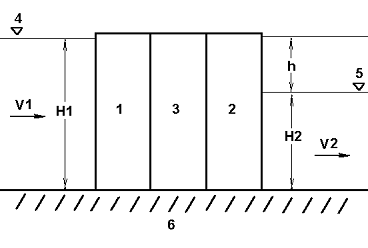Explanations for Fig. 1:

1 - Working parts of the inflowing stream of water;

2 - Working parts of the outflowing stream of water;

3 - Working parts ensuring positive feedback between the inflowing and outflowing streams of water;

4 - Mark showing the level of the inflowing stream of water;

5 - Mark showing the level of the outflowing stream of water;

6 - Channel bed

H1 – Actual depth of the inflowing stream of water

H2 – Depth of the outflowing stream of water

V1 – Velocity of the inflowing stream of water

V2 – Velocity of the outflowing stream of water

h – Drop between the levels of the inflowing and outflowing streams of water

The device works based on the following principle:

The working parts of the inflowing stream 1 extract part of the kinetic energy from the stream and transmit it - with the help of the positive feedback 3 - to the working parts of the outflowing stream 2, which give the outflowing stream additional acceleration.

Because the amount of water entering the device is equal to the amount of outflowing water, and the speed of the outflowing stream is higher than that of the inflowing stream, then the  sectional  area of the outflowing stream will be less than that of the inflowing stream.

Therefore, its depth H2 will be less than the depth of the inflowing stream H1 by the value h. As a result of this, potential energy appears between the different levels of the inflowing and outflowing streams.

The device’s energy balance is as follows:

E = Ep1 + Ek1 – Ek2

The total output of energy from the device is equal to the potential energy of the difference between the marks plus the kinetic energy of the inflowing stream and minus the kinetic energy of the outflowing stream.  After omitting all the computations, we have: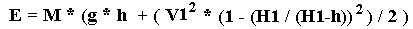orwhere M is the weight of the water entering the device in a unit of time, which is equal to the density of water multiplied by the active area of the inflowing stream and multiplied by its velocity.

Then the most interesting aspect occurs. It can be seen that the left side of the equation, which is in brackets, will increase in a linear fashion when it depends on h or in a hyperbola when it depends on V2, whereas the right part will decrease, and in a parabola at that. Which side will gain the upper hand?

Let us plot a graph showing energy’s dependence on the drop between the levels h. The graph will be plotted to show the various levels of the inflowing stream’s velocity V1 after designating it as a constant.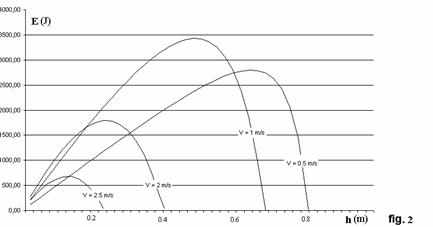It is a paradox! The graph showing the energy’s dependence on the drop between the levels h has an extremum. On the rising branch of the graph, the energy balance will be positive (the power factor > 1), i.e. the extracted potential energy will be mostly expended as kinetic energy on speeding up the outflowing stream, and the device will self-accelerate until it reaches the maximum.

The energy produced by the device at this point will be several times the kinetic energy of the inflowing stream - and under certain conditions, tens and even hundreds of times!

The speed of the outflowing stream will be significantly higher (2 to 3 times as higher at times) than the speed of the inflowing stream. Therefore, the kinetic energy of the outflowing stream is 4 to 9 times the kinetic energy of the inflowing stream.

Furthermore, the graphs show that that not everything appears to be quite right with the inflowing speed. It also has an extremum. To see this better, let us plot a 3D diagram.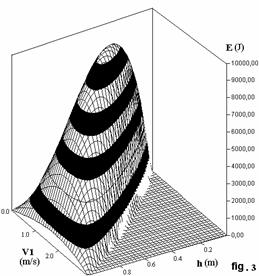Isn’t it beautiful?!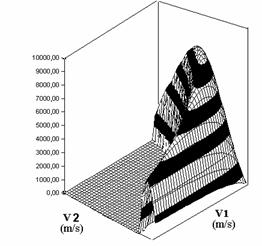This is the dependence on the outflowing speed.

However paradoxical this may seem at first glance, but the diagrams show there is an optimal speed for the inflowing stream. When it is exceeded, the device’s power capacity will sharply fall. This is due to the fact that a significant amount of energy needs to be spent on speeding up a stream that is flowing fast already.

Therefore, it can be seen that the device can create a column of water for itself and is able to extract the potential energy from an object (from a stream of water in this case) without the expenditure of external energy.

Does this not remind you of something? People who are knowledgeable about physics will immediately exclaim: “Why, this is Maxwell’s demon!” Indeed! The much-discussed Maxwell’s demon that has thus far been elusive. Many people will say that Maxwell proposed his “demon” for thermodynamics, and here you are dealing with hydrodynamics. Yes, but this does not change the essence of the matter – we can extract from an object (in this case, a flow of liquid) the potential energy that cannot be extracted in normal conditions. And we can extract it without spending anything (without even building a dam!) at that. It is true that not all of the potential energy can be extracted. Firstly, the depth of the outflowing stream is not equal to zero. Secondly, part of the potential energy extracted transforms into additional kinetic energy splashed out with this flow. This energy is actually even greater than the kinetic energy of the inflowing stream. However, this is the reward we should give the “demon” so that it agrees to work for us.  As you see, the “demon” also “wants to eat”.

The question may arise: “How does the outflowing stream, which has a shallower depth, interact with the water flow around it, which has a normal constant depth?” Here we have to recall that the velocity of the outflowing stream is higher than that of the surrounding medium and this creates what is called in hydraulics “hydraulic jump”, which equalises the discrepancy between the kinetic and potential energies of the two flows. This “jump” is in essence surf, a vortex in the flow.

The conclusions to be drawn from what has been outlined above cannot be overestimated. In nature there exists a process which makes it possible to extract energy which it was impossible to extract in the past from any object - and this process has been discovered! This is the principle of positive feedback that makes it possible to transfer energy between different flows of energy sources. There is the possibility of extracting free, environmentally-pure energy from the environment, which was predicted by the great Scottish physicist James Maxwell back in 1871 in the form of a jokey demon. Maybe it was precisely because of this that it was always regarded as nothing more than a joke by the great scientist. Or is it reality indeed?

It is not quite clear yet how it works with thermodynamics and aerodynamics, but because this process exists in hydrodynamics it should also exist in any other branch of physics. There are some developments in thermodynamics and aerodynamics already. Even if this process is not found for them in the near future and it drags on for decades, then at least applying its hydrodynamic interpretation is already promising mankind huge dividends in the form of free energy and an uncontaminated atmosphere

In the next article, we will discuss what seems as the utopian idea (possibility) of using this principle of extracting energy on cars, and a hypothetical engine for them.

German  Treshalov is a hydro-energy engineer, head of the Engineering Research Group to develop alternative sources of energy.

Translated by Ascar  Jumanov,   Joanna Lillis
01.08.06

Note: applications have been submitted to obtain international patents for the methods of extracting energy and designing devices that use this method and constructing such devices.

V. Brodyanskiy “Exergetic analysis. Energy: the Problem of Quality”, Nauka I Zhizn, No 3, 1982
N. Shchapov “Turbine Equipment for Hydropower Stations”, Gosenergoizdat, 1961
N. Gulia “In Search of an Energy Capsule”, a web publication
E. Oparin “Physical Foundations of Fuelless Power-Engineering. The Limitation of the Principle of Entropy Increase”, Moscow, URSS, 2004
L. Landau, A. Kitaygorodskiy “Physics for Everyone”, Nauka, 1974

EP2019202
METHOD OF EXTRACTING ENERGY FROM STREAM OF FLOWING MEDIUM

EC:   F03B13/00; F03B13/08; (+1)  IPC:   F03B13/00; F03B13/00
2009-01-28

Abstract -- Die Erfindung betrifft ein Verfahren zur Gewinnung von Energie aus einem freiströmenden, horizontalen Strom eines Fliessmediums und ein Berechnungsverfahren für energieerzeugende Maschinen, die nach dem erstgenannten Prinzip arbeiten. Das unterscheidende Merkmal des beschriebenen Verfahrens besteht in der Verwendung einer Rückkopplung zwischen der Energie des einströmenden Stroms und der Energie des herausströmenden Stroms eines Fliessmediums. Die Rückkopplung wird durch besonders entwickelte Hydraulikmaschinen gewährleistet. Eine schematische Zeichnung einer derartigen Maschine dient zur Erläuterung dieses Verfahrens.Your Support Maintains this Service --